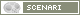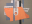OpenMusic DocumentationOM 6.6 User Manual > Visual Programming II > Iterations: OMLoop > Accumulators > Sum
page précédentepage suivante

Sum : Calculating Sums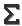Sum adds the successive input values.

Inputs, Outputs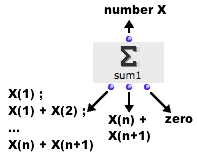Sum has one input and three outputs :"what" : collects the successive values coming from other boxes. first output :triggers the collection of a new value, and the addition of this value to a sumreturns the current value of the sumsecond output : returns the value of the current state of sumthird output : resets sum to zero.

Examples

Calculating a Sum from a List
 The components of a list are returned successively by listloop to sum. At each step of the loop, eachtime triggers and prints the sum of the components.The state of sum at the end of the loop, that is, the sum of all the components of the list, is returned by finally.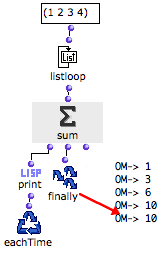Calculating the Sum of N Random Numbers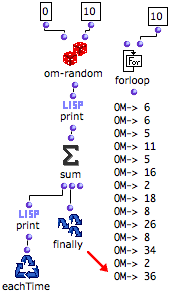We now want to calculate the sum of 10 random numbers between 1 and 10. The number of loops is defined by forloop. At each step : a random number is returned by om-random and printedthe sum of the successive is returned by sum and printed.After ten loops, the iteration is stopped by forloop. Finally returns the sum of the whole set of numbers returned by om-random.
page précédentepage suivante
A propos...(c) Ircam - Centre Pompidou